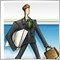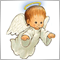# PlotIndexSetInteger(0,PLOT_SHOW_DATA,false) , its for mq5 . what is Equal for mq4 ?4796

PlotIndexSetInteger(0,PLOT_SHOW_DATA,false) , its for mq5 . what is Equal for mq4 ?3024

PlotIndexSetInteger(0,PLOT_SHOW_DATA,false) , its for mq5 . what is Equal for mq4 ?

There isn't equal function in MQL4. But if you don't want to draw a buffer in MQL4 you can use something like this:
```SetIndexBuffer(bufferIndex,buffer,INDICATOR_CALCULATIONS);
SetIndexStyle(bufferIndex,DRAW_NONE);
SetIndexLabel(bufferIndex,NULL);```4796

Petr Nosek:
There isn't equal function in MQL4. But if you don't want to draw a buffer in MQL4 you can use something like this:

thank you.38676

Petr Nosek:
There isn't equal function in MQL4. ...

Not quite true. Equivalent is :

```   SetIndexLabel(0,NULL);
```4796

Alain Verleyen:

Not quite true. Equivalent is :

YES.thank you